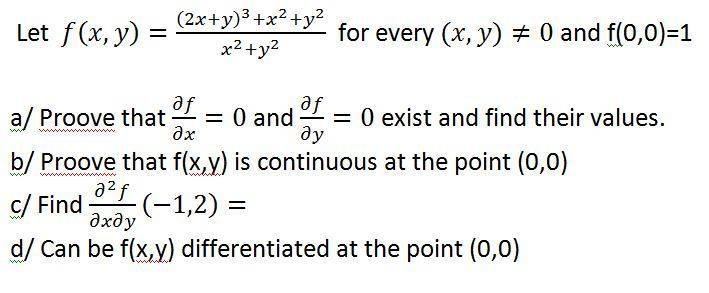# Continuity of a two-variable function

## Homework Statement## Homework Equations

My main problem is connected with b/ and d/. I found a formula involving the norm of the function, but I'm not sure if it's a good idea using it.

## The Attempt at a Solution

I can prove that a function is not continuous by finding different values for x and y for which the limit at the point is approaching different values, but have no idea how to prove that it is continuous.

Last edited:

## Answers and Replies

LCKurtz
Science Advisor
Homework Helper
Gold Member
Hint for (b): Look at

$$\left| \frac{(2x+y)^3+x^2+y^2}{x^2+y^2} - 1\right|$$

Simplify it and change it to polar coordinates. Think about $r\rightarrow 0$.

Thank you